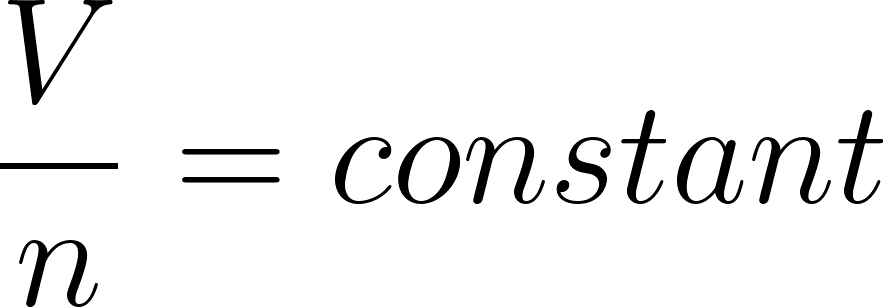×
Get Full Access to Introductory Chemistry - 5 Edition - Chapter 11 - Problem 13q
Get Full Access to Introductory Chemistry - 5 Edition - Chapter 11 - Problem 13q

×ISBN: 9780321910295 34

## Solution for problem 13Q Chapter 11

Introductory Chemistry | 5th Edition

• Textbook Solutions
• 2901 Step-by-step solutions solved by professors and subject experts
• Get 24/7 help from StudySoup virtual teaching assistantsIntroductory Chemistry | 5th Edition

4 5 1 248 Reviews
21
5
Problem 13Q

PROBLEM 13Q

What is Avogadro's law? Explain Avogadro's law from the perspective of kinetic molecular theory.

Step-by-Step Solution:

Problem 13Q

What is Avogadro's law? Explain Avogadro's law from the perspective of kinetic molecular theory.

Step by Step Solution

Step 1 of 2

According to the Avagadro’s law , volume of a given amount of a gas at constant temperature and pressure is directly proportional to the number of moles of the gas.Here,

V = Volume

n = Number of moles of gas

From the law,

As the number of moles of the gas increases , volume of the gas increases.

As the number of the gas decreases , volume of the gas decreases.

Step 2 of 2

##### ISBN: 9780321910295

Unlock Textbook Solution